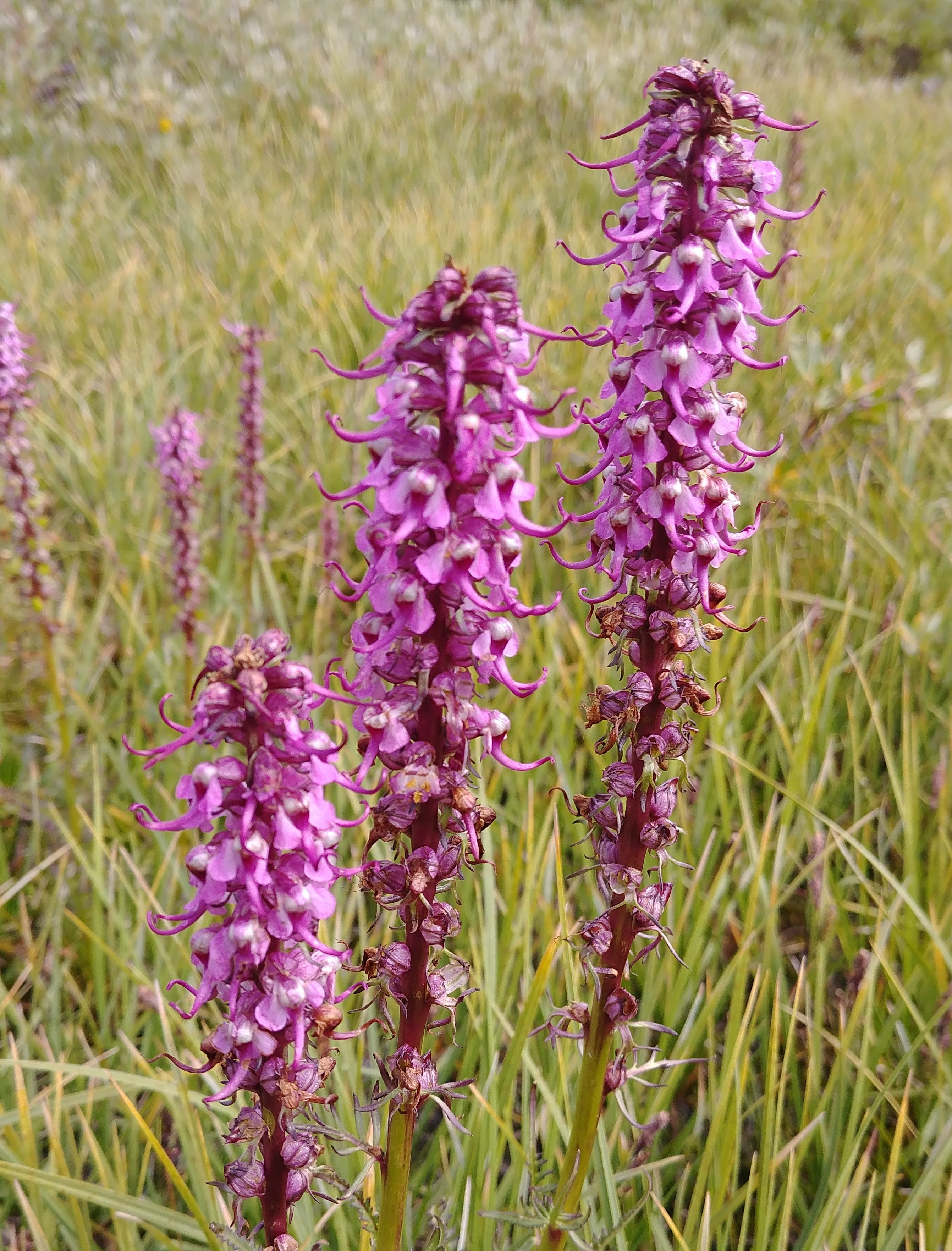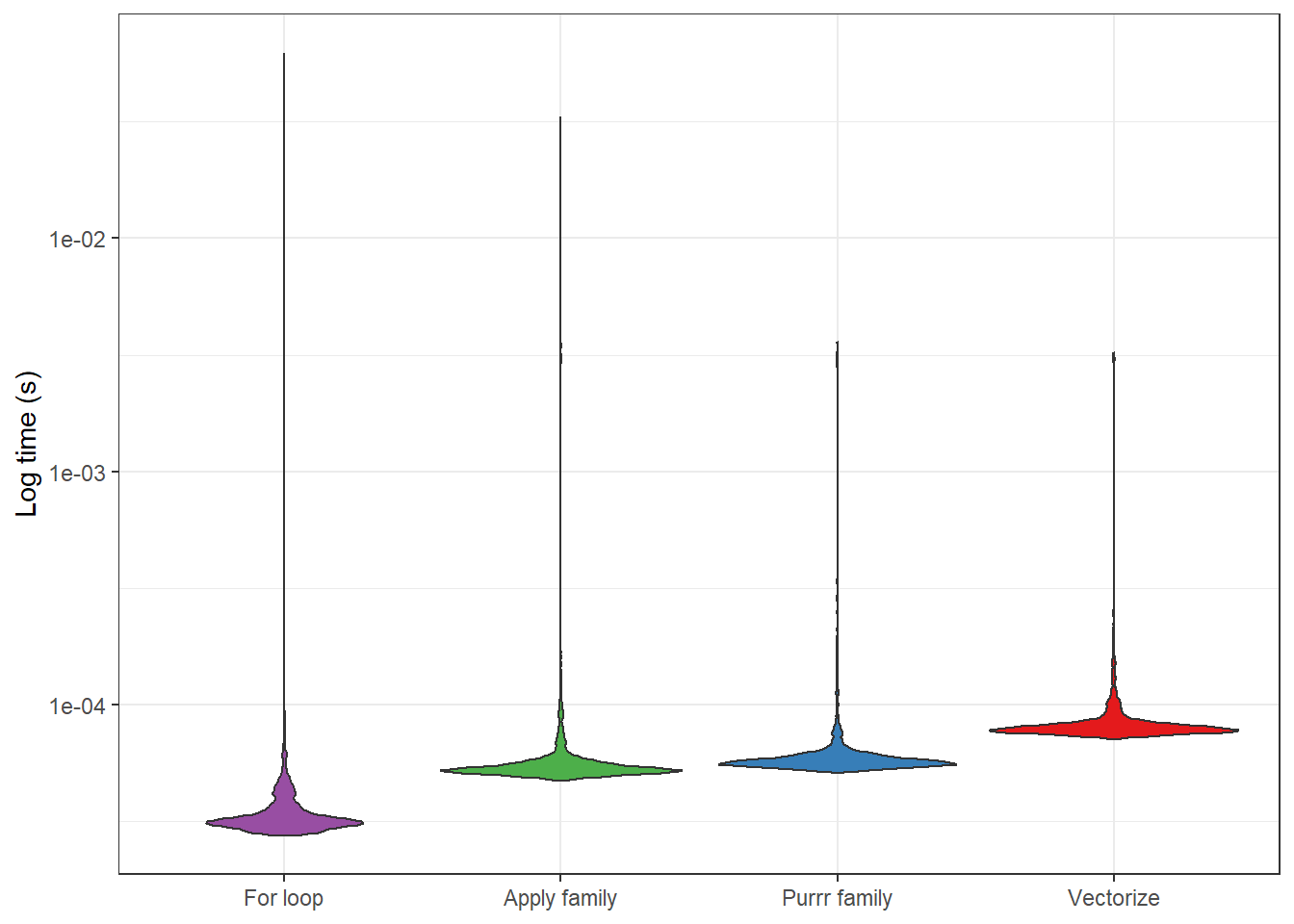# Wetlands and landscapes

Data science, water, isotopes, and ecosystems

## A brief visualization of R's distribution functions, focusing on the normal distribution

The intent of this post is to provide a visual intuition of the various distribution functions in R, using the normal distribution as an example.When I first started playing with the distribution functions in R I would always get confused as to what the different name combinations meant. For example, the differences between `rnorm()`, `dnorm()`, `dt()`, `rbinom()` were all pretty mysterious to me. Now I understand these names are composed of two parts:

## Base R, the tidyverse, and data.table: A comparison of R dialects to wrangle your dataSynopsis: Below are a number of examples comparing different ways to use base R, the tidyverse, and data.table. These examples are meant to provide something of a Rosetta Stone (an incomplete comparison of the dialects, but good enough to start the deciphering process) for comparing some common tasks in R using the different dialects. The examples start fairly simply and get progressively harder. I provide some additional information along the way, in case folks are new to R or programming more…

## Vectorize in R

Letting the Vectorize function do the heavy-lifting when it comes to applying complicated control flows.One of the cool things about R is that it is a vectorized language. This means that you can apply a vector (i.e., a series of values) to functions like `sqrt` and `log` without the need to write a for loop. While I was naive to how useful this was when learning R, in retrospect, I now very much appreciate this behavior.